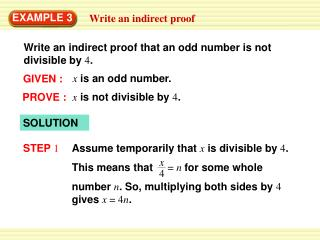DownloadDownload PresentationEXAMPLE 3

# EXAMPLE 3

Télécharger la présentation## EXAMPLE 3

- - - - - - - - - - - - - - - - - - - - - - - - - - - E N D - - - - - - - - - - - - - - - - - - - - - - - - - - -
##### Presentation Transcript

1. x Assume temporarily that xis divisible by 4. This means that = n for some whole number n. So, multiplying both sides by 4 gives x = 4n. 4 EXAMPLE 3 Write an indirect proof Write an indirect proof that an odd number is not divisible by 4. xis an odd number. GIVEN : xis not divisible by 4. PROVE : SOLUTION STEP 1

2. If xis odd, then, by definition, xcannot be divided evenly by 2. However, x = 4nso = = 2n. We know that 2nis a whole number because nis a whole number, so x can be divided evenly by 2. This contradicts the given statement that xis odd. x 2 4n 2 EXAMPLE 3 Write an indirect proof STEP 2 STEP 3 Therefore, the assumption that xis divisible by 4 must be false, which proves that xis not divisible by 4.

3. Suppose you wanted to prove the statement “If x + y = 14 and y = 5, then x = 9.” What temporary assumption could you make to prove the conclusion indirectly? How does that assumption lead to a contradiction? Assume temporarily that x = 9; and y = 5 are given since x + y = 14 Therefore, letting x = 9 leads to the contradiction 9 + 5 = 14. for Example 3 GUIDED PRACTICE 4. SOLUTION STEP 1 STEP 2